You are here : Main projects | The Zeus Tesla Coil | 2. Theory of operation

The Zeus Tesla Coil
September 2011

# 2.  Theory of operation

In this chapter, we will discuss the theory of operation of Tesla coils in a general way. For the moment, let us introduce this short definition: "A Tesla coil is a device producing a high frequency current, at a very high voltage but of relatively small intensity". Basically, it works as a transformer and as a radio antenna, even if it differs radically from these.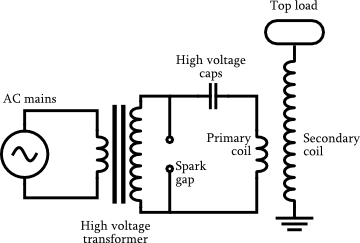Figure 2.1 — Basic electric schematic of a Tesla coil.
[Image credits: Wikipedia]

Note : If you remember your Physics 101 well enough, you may directly commence at the Tesla coil operation section. The first sections are indeed mostly reminders.

## 2.1   Reminder of the basics

### 2.1.1   Resistor

A resistor is a component that opposes a flowing current. Every conductor has a certain resistance. If one applies a potential difference V at the terminals of a resistor, the current I passing through it is given by :

I = V/R

This formula is know as Ohm’s Law. The SI unit of resistance is the Ohm, noted Ω. One can show that the power P (in J/s) dissipated due to a resistance is equal to

P = VI = RI2

### 2.1.2   Capacitor

A capacitor is a component that can store energy in the form of an electric field. Less abstractly, it is composed in its most basic form of two electrodes separated by a dielectric medium. If there is a potential difference V between those two electrodes, charges will accumulates on those electrodes : a charge Q on the positive electrode and an opposite charge -Q on the negative one. An electrical field therefore arises between them. If both of the electrodes carry the same amount of charge, one can write

Q = CV

where C is the capacity of the capacitor. Its unit is the Farad, noted F. The energy E stored in a capacitor is given by

E = QV/2 = CV2/2

where one can note that the dependence in the charge Q shows that the energy is indeed the energy of the electric field. This corresponds to the amount of work that has to be done to place the charges on the electrodes.

### 2.1.3   Inductor

An inductor stores the energy in the form a magnetic field. Every electrical circuit is characterised by a certain inductance. When current flows within a circuit, it generate a magnetic field B that can be calculated from Maxwell-Ampère's law :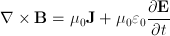where E is the electric field and J is the current density. The auto-inductance of a circuit measures its tendency to oppose a change in current : when the current changes, the flux of magnetic field ΦB that crosses the circuit changes. That leads to the apparition of an "electromotive force"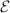that opposes this change. It is given byThe inductance L of a circuit is thus defined as :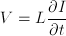where I(t) is the current that flows in the circuit and V the electromotive force (emf) that a change of this current will provoke. The inductance is measured in henrys, noted H.

The energy E stored in an inductor is given by:

E = LI2/2

where the dependence in the current I shows that this energy originates from the magnetic field. It corresponds to the work that has to be done against the emf to establish the current in the circuit.

### 2.1.4   Impedance

The impedance of a component expresses its resistance to an alternating current. This quantity generalizes the notion of resistance. Indeed, when dealing with alternating current, a component can act both on the amplitude and the phase of the signal.

#### Expressions for alternating current

It is convenient to use the complex plane to represent the impedance. The switching between the two representations is accomplished by using Euler's formula. Let's note that the utilization of complex numbers is a simple mathematical trick, as it is understood that only the real part of these quantities is meaningful. We are now given an expression of the general form of the voltage V(t) and current I(t) :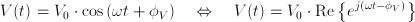where V0 and I0 are the respective amplitudes, ω = 2πν is the angular speed (assumed identical for both quantities) and φ are the phases.

#### Definition of impedance

The impedance, generally noted Z, is formed of a real part, the resistance R, and an imaginary part, the reactance X :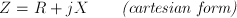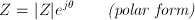where j is the imaginary unit number, i.e. j² = -1, θ = arctan(X/R) is the phase difference between voltage and current, and |Z|=(R²+X²)1/2 is the euclidean norm of Z in the complex plane.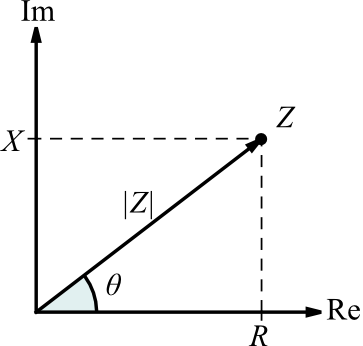Figure 2.2 — The impedance Z plotted in the complex plane.
[Image credits: Wikipedia]

At this point, we can generalize Ohm's law as the following

V(t) = Z I(t)

When the component only acts on the amplitude, in other words when X = 0, the imaginary part vanishes and we find Z = R. We therefore recover the behaviour of a resistor. The component is then said to be purely resistive, and the DC version of Ohm's law previously mentioned applies. When the component only acts on the phase of the signal, that is when R = 0, the impedance is purely imaginary. This translates the behaviour of "perfect" capacitors and inductors.

#### Impedance formulas

We can give a general formula for the impedance of each type of component.

Component Impedance Effect on an alternating signal
Resistor Z = R Diminution of amplitude (current and tension).
Capacitor Z = (jCω)-1 Tension has a π/2 delay over current.
Inductor Z = jLω Current has a π/2 delay over tension.

These formulas are easily recovered from the differential expressions of these components. For every combinations of components, one can calculate the phase difference between current and voltage by vector-adding the impedances (for example, in an RC circuit, the phase difference will be less than π/2). Finally, it is good to keep in mind that any real-life component has a non-zero resistance and reactance. Even the simplest circuit, a wire connected to a generator has a capacitance, an inductance and a resistance, however small these might be.

## 2.2   LC circuit

An LC circuit is formed with a capacitor C and an inductor L connected in parallel or in series to a sinusoidal signal generator. The understanding of this circuit is at the very basis of the Tesla coil functioning, hence the following analysis. The primary and secondary circuits of a Tesla coil are both series LC circuits that are magnetically coupled to a certain degree. We will therefore only look at the case of the series LC circuit.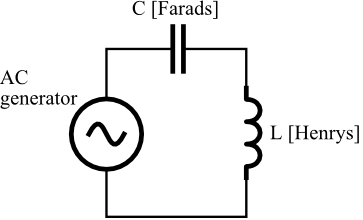Figure 2.3 — Schematic of a series LC circuit.

### 2.2.1   Analysis

Using Kirchoff's law for current, we obtain that the current in the inductor and the current in the capacitor is identical. We now use Kirchoff's law for voltage, which sates that the sum of the voltages across the components along a closed loop is zero, to get the following equation :

Vgen(t) = VL(t) + VC(t)

For the inductor, we use the expression for V previously found and express the time derivative of current in terms of the charge by I = dQ/dt. We find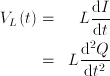Now for the capacitor, we isolate the charge Q in the relation previously found giving the voltage across a capacitor and get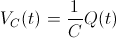Plugging these two results in the equation for Vgen, we obtain (using Newton's notation) :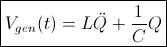This equation describes an (undamped) harmonic oscillator with periodic driving, just like a spring-mass system ! The inductor is assimilated to the "mass" of the oscillator : a circuit of great inductance will have a lot of "inertia". The "spring constant" is associated with the inverse of the capacitance 1/C (this is the reason why 1/C is seldom called the "elastance").

#### Homogeneous solution

The homogeneous equation, i.e. without independent term (representing the generator, in this case) is the following :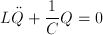This equation corresponds to a real situation: the capacitor holds a certain charge (or the inductor has a certain current, or both) and we let the system evolute freely. The mechanical analogy with the spring-mass system correspond to an initial state where the spring is tense (or the mass moving, or both) and when no other force interacts. We find the solution this equation with the characteristic polynomial method. We obtain a sum of complex exponentials, which is of course an oscillating solution as expected.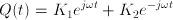where we noted ω = (LC)-1/2 (K1 and K2 are just integration constants). Setting Q(0) ≡ Q0 and Q'(0) = I(0) ≡ 0, which corresponds to the initial condition where the capacitor is fully loaded and no current initially flows in the inductor, we get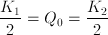We now take the real part of Q(t), but thanks to what we just found, the solution is already real :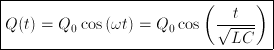We now find the current flows through the inductor by time-derivating the above expression for the charge stored in the capacitor :The next figures give a good intuition of what actually happens in the circuit. We look at the voltage V(t) between the leads of the capacitor as well as the current I(t) running into the inductor (which is the same in the entire circuit, as previously stated). For convenience, we put all the constants Q0, L, and C to unity.

Figure 2.4 — Left: LC circuits with voltmeter and ammeter. Right: Plot of tension in current versus time.

Step 0:   At the initial instant, the capacitor is fully charged and no current flows. Immediately after, the capacitor begins to discharge: the voltage decreases as a current gradually appears in the circuit.

Step 1:   When the capacitor is totally discharged, the voltages at its leads is zero. If there was no inductance, things would stop right here. However, the inductor opposes this brutal drop of current by generating an electromotive force that will keep the current going. In this way, the current gradually decreases (instead of stopping abruptly) and recharges the capacitor on the way (with an opposite polarity).

Step 2:   The capacitor is fully charged again, with opposite polarity now, and the current has fallen to zero. However the charges stored in the capacitor are willing to neutralize themselves : a current will reappear while the voltage is decreasing again.

Step 3:   The capacitor is "empty" again (zero voltage), but the inductor prevents the current from stopping abruptly. This current will now recharge the capacitor with reversed polarity...

Step 4:   ... and the situation is exactly like it was at the initial instant.

# N.B.

Let us stress at this point that the system naturally oscillates at a certain resonant angular speed ω = (LC)-1/2, which is univoquely determined by the inductance and the capacitance of the circuit.

#### General solution

We reconsider equation for Vgen with its independent term, where we explicitly state the fact that the generator produces a sinusoidal voltage of amplitude, say, V0 and of pulsation Ω: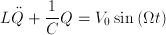We will find a specific solution to obtain the general solution.

If ω ≠ Ω.   We make an educated guess about a specific solution Qp by stating it has the following form: Qp = Asin(Ωt - φ), with amplitude A and phase φ to be determined. We derivate this guessed solution twice and inject it straight into our starting equation :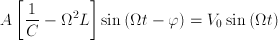Equating amplitude and phase into the two parts of this equality, we deduce :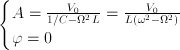The specific solution is therefore :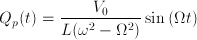We can now find the general solution by adding the specific solution to the homogeneous solution previously found.The current is :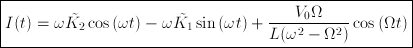where the integration constants can be found in terms of the initial conditions. We see that we still have sinusoidal functions. If ω is close to Ω, the oscillations will be of great amplitude because of the "new" term, but will remain bounded. When ω is very far from Ω, the new term becomes negligible.

If ω = Ω.   Here, the specific solution is more difficult to find. Let us rewrite our starting (inhomogeneous) equation in a more convenient way :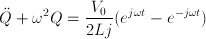Here, we'll guess that the specific solution has the following form ; Qp(t) = (A+ + B+t)ejωt + (A- + B-t)e-jωt. This makes sense physically, as we expect the voltage and current to have amplitudes that increase with time. We rewrite it in a more condensed manner : Qp(t) = (A + Bt)ekt with k = ±jω. The second time-derivative yields d2Qp/dt2(t) = [k2(A + Bt) + 2kB]ekt. We plug these expression into our equation for Q in order to refine them :We compare the two side of the equality as before. When k = jω, we find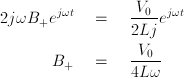When k = -jω,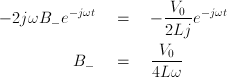We have therefore found that B+ = B- ≡ B. And as we have found no constraints on the A's, we set them equal to zero. We have now obtained our specific solution :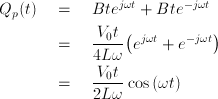The general solution for the voltage is therefore :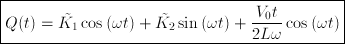and the current is :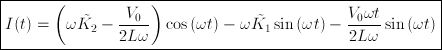where again the integration constants can be found in terms of the initial conditions. In this case, we see we're no longer dealing with simple oscillations. The last term of the solution indicates that the amplitude of the voltage and current will increase at each cycle, and grow to infinity as t goes to infinity. Note that there is no energy conservation violation : all that happens is that -in a perfect oscillator driven at its own resonant frequency- all the injected energy remains in the system.

# N.B.

The system we just finished studying is very simple, but it already displays the exploding-voltage-and-current behaviour of a Tesla coil...

### 2.2.2   Resonant frequency

In our analysis of the LC circuit, we found that the oscillations of current and voltage naturally occurred at a precise angular speed, univoquely determined by the capacitance and inductance of the circuit. Without other effects, oscillations of current and voltage will always take place at this angular speed.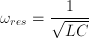It is called the resonant angular speed. We can check that it is dimensionally coherent (its units are s-1). It is however much more common to talk about resonant frequency, which is just a rescale of the angular speed :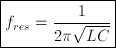It is no less important to observe that, at the resonant angular speed, the respective reactive parts of an inductor and a capacitor are equal (in absolute value) :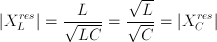When there is a sinusoidal signal generator, we also saw that if its frequency is equal to the resonant frequency of the circuit it drives, current and voltage have ever-increasing amplitudes. Of course, this doesn't happen if they are different (the oscillation remain bounded).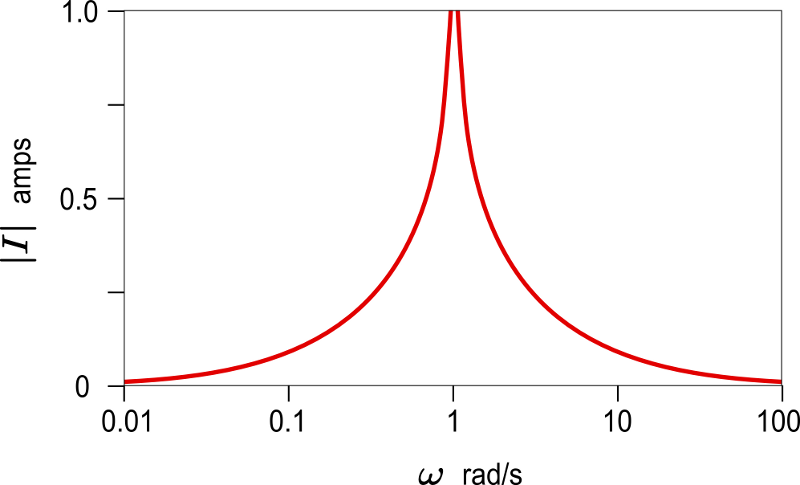Figure 2.5 — Amplitude of the current plotted against the driving frequency (all constants normalised). [Image credits: Wikipedia]

At low driving frequencies, the impedance is mainly capacitive as the reactance of a capacitor is greater at low frequencies. At high frequencies, the impedance is mainly inductive. At the resonant frequency, it vanishes, hence the asymptotic behaviour of the current. However, in a real circuit, where resistance is non-zero, the width and height of the "spike" plotted her above are determined by the Q factor, which we'll talk about later. The fact that driving an (R)LC circuit at its resonant frequency causes a dramatic increase of voltage and current is crucial for a Tesla coil. But it can also be potentially harmful for the transformer feeding the primary circuit. These practical considerations will be addressed in the next chapter.

### 2.2.3   Impedance

We'll have to calculate the impedance of the LC circuits inside the Tesla coil. Let us recall that the capacitor and the inductor are connected in series on the generator. The total impedance is therefore equal to the sum of the impedances of each component.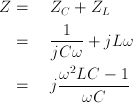We see that, at the resonant pulsation, ω2LC = 1 and the impedance vanishes. A series LC circuit placed in series on a wire will therefore act as a band-pass filter. Similarly, a parallel LC circuit will act as a band-stop filter when connected in series on the wire. There are many other possibilities for filters, but this is not the scope of the present document.

## 2.3   RLC circuit

So far, we have neglected all dissipating forces. This is why we had to cope with things like infinite currents and voltages. But every real circuit has a non-zero resistance, and we'll see that taking this into account will erase away these divergent behaviours. An RLC circuit is an LC circuit on which a resistance was added. This kind of circuit can take multiple forms. The resistance (VR(t)=RQ'(t)) dissipates the circuits energy as heat, whatever the direction of the current. It therefore acts like a friction force. Without a generator, current and voltage will gradually decrease as they oscillate (the circuit is said to be underdamped) or, if the resistance is high enough, no oscillation will take place (overdamped circuit). In the underdamped case, the graph of current or voltage is a sine wave whose amplitude decreases exponentially with time. If there is a driving force, the resistance will prevent the system from having oscillations of ever-increasing amplitude. And finally, instead of a spike, the RLC circuit has a bandwidth.

Figure 2.6 — Left: Schematic of a series RLC circuit. Right: Amplitude of the current vs driving frequency for an RLC circuit (all constants normalised). [Right image credits: Wikipedia]

### 2.3.1   Analysis

Let's make a quick mathematical analysis of the series RLC circuit with a sinusoidal voltage generator, like the one on the previous figure. We'll treat only the underdamped case (0 < R/2L < (LC)-1/2), as it is the one relevant to Tesla coil. The results for the underdamps and critically damped cases can be found on the PDF file. This gets a bit dirty, but the results we'll find are worth the work. The equation describing this system is different from the equation of the LC circuit because of the added resistor R. The tension across the leads is given by Ohm's law V = RI. Using Kirchoff's law for currents, we know that the current I(t) is identical in the entire loop. We then have :or in a equivalent way :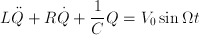where we made use the relation I = dQ/dt.

#### Homogeneous solution

We first look at the situation where there's no driving force. We begin by setting the two following quantities, which both have the dimensions of an angular speed :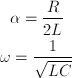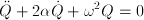By resolving this equation with the characteristic polynomial method, one finds :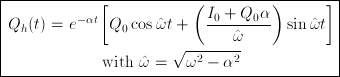where Q0 and I0 respectively represent the charge and the current at the initial moment t = 0. In this free regime, we see that the system carries out oscillations whose amplitude exponentially decrease with time, conversely to the LC circuit where the oscillation amplitude stays constant. We also remark that the natural pulsation of the oscillations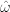(mind your hats) has "shifted" from the LC's one, which was ω = (LC)-1/2. Finally, we notice that at the limit where the attenuation term α goes to zero, we recover the solution for the LC circuit. One finds the current I(t) by derivating the previous expression with respect to time.

#### General solution

One can verify that the following function is indeed a particular solution to our starting equation :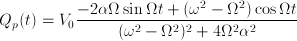The general solution is therefore :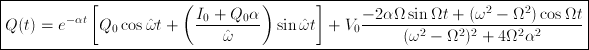Here we see that the term 4Ω2α2 prevents the asymptotic behaviour encountered in the LC circuit when Ω = ω : Oscillations remain bounded no matter what in an RLC circuit. This explains the differences between Figs. 2.6 and 2.5. An important thing to note is that, beside the frequency of the free oscillations of an RLC circuit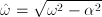being different from the LC circuit's, the resonant frequency in driven regime remains the same : the last term of Q(t) is maximal when Ω = ω and not. This quite remarkable fact explains why the results on resonant frequency derived for the unrealistic LC circuit still holds for Tesla coil circuits.

### 2.3.2   Impedance

To find its impedance, we just have to add the value of the resistance R to the impedance of the LC circuit. While the later had a purely imaginary impedance, the RLC circuit has a real part as well :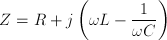### 2.3.3   Q factor

The Q factor (for quality) is a dimensionless quantity which characterises every RLC circuit, or more generally, every damped oscillator. It measures the narrowness of the bandwidth : a large Q factor denotes a spiky bandwidth. More precisely, the Q factor represents the ratio between the energy stored in the circuit and the energy dissipated during the oscillations. This can also be expressed in power terms :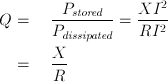where X is the reactance of the inductor/capacitor at the resonant frequency. For a RLC circuit, this becomes :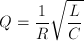One can also give a definition of the bandwidth Δf as a function of the Q factor :It is then clear why this quantity is called the quality factor : an RLC circuit of "great quality" is strongly underdamped and will therefore dissipate very little energy per cycle. Moreover, its bandwidth will be very narrow, which is desirable, for example, in a radio receiver.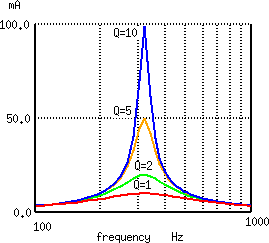Figure 2.7 — Effect of the Q factor on the bandwidth size.
[Image credits : All About Circuits]

## 2.4   Tesla coil operation

We are now ready for the theory of operation of spark gap Tesla coils properly said. We'll first describe how a cycle works, then compare the Tesla coil to transformers and quarter-wave antennas. A last section will treat the mathematical analysis of inductively coupled LC circuits, proving some assumptions of the previous sections along the way.

### 2.4.1   Description of a cycle

This is where the fun begins. This section shall cover the complete theory of operation of a conventional Tesla coil. We will consider that the primary and secondary circuits are RLC circuits with low resistance, which accords with reality. We consider a slightly modified version of figure 2.1 to illustrate our explanations. For the aforementioned reasons, internal resistance of the component aren't represented. We will also replace the current-limited transformer. This has no impact regarding pure theory.

Note that some parts of the secondary circuit are drawn in dotted lines. This is because they are not directly visible on the apparatus. Regarding the secondary capacitor, we'll see that its capacity is actually distributed, the top load only being "one plate" of this capacitor. Regarding the secondary spark gap, it is shown in the schematic as a way to represent where the arcs will take place.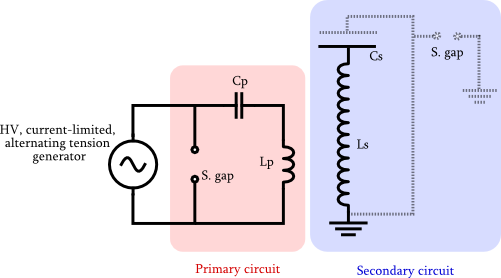Figure 2.8 — Schematic of the basic features of a Tesla coil.

#### Charging

This first step of the cycle is the charging of the primary capacitor by the generator. We'll suppose its frequency to be, say, 50 Hz. Because the generator is current-limited, the capacity of the capacitor must be carefully chosen so it will be fully charged in exactly 1/100 seconds. Indeed, the voltage of the generator changes twice a period, and at the next cycle, it will re-charge the capacitor. Note that it is then charged with opposite polarity, but this changes absolutely nothing about the operation of the Tesla coil.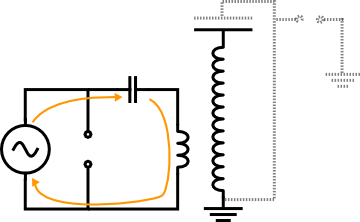Figure 2.9 — The generator charges the primary caps.

#### Oscillations

When the capacitor is fully charged, the spark gap fires and therefore closes the primary circuit. Knowing the intensity of the breakdown electric field of air, the width of the spark gap must be set so that it fires exactly when the voltage across the capacitor reaches its peak value. The role of the generator ends here (the impedance of the spark gap is far lower than the transformer impedance).

We now have a fully loaded capacitor in an LC circuit! Current and voltage will thus oscillate at the circuits resonant frequency, as it was demonstrated in the previous page. This frequency is very high compared to the mains frequency, generally between 50 and 400 kHz.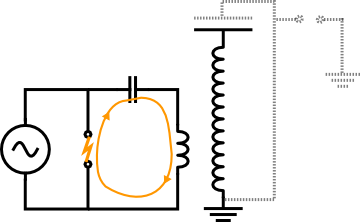Figure 2.10 — The spark gap fires, and the current oscillates in the primary circuit.

The primary and secondary circuits are magnetically coupled. The oscillations taking place in the primary will thus induce an electromotive force in the secondary. As the energy of the primary is dumped into the secondary, the amplitude of the oscillations in the primary will gradually decrease while those of the secondary will amplify. This energy transfer is done through magnetic induction. The coupling constant k between the two circuit is purposefully kept low, generally between 0.05 and 0.2. Several oscillations will therefore be required to transfer the totality of the energy. We will come back to the influence of coupling shortly.

Figure 2.11 — Left: Oscillations in the primary will induce and emf in the secondary, which will also oscillate. Right: The electromotive force induced by the oscillations in the primary can be seen as an AC generator (whose amplitude depends on time) as shown here, although that is not strictly correct.

The oscillations in the primary will thus act a bit like an AC voltage generator placed in series on the secondary circuit. To maximise the voltage in the secondary, it is intuitively clear that both circuits must share exactly the same resonant frequency, as it will be demonstrated below.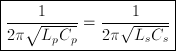This will allow the voltage in the secondary to increase dramatically, as we saw in equations describing a harmonically driven LC circuit. This is called the resonant rise. The voltage becoming rapidly enormous, generally several hundreds of thousand of volts, sparks will form at the top load. The following diagrams show the general waveforms (in arbitrary units) of the oscillations taking place in the primary and secondary.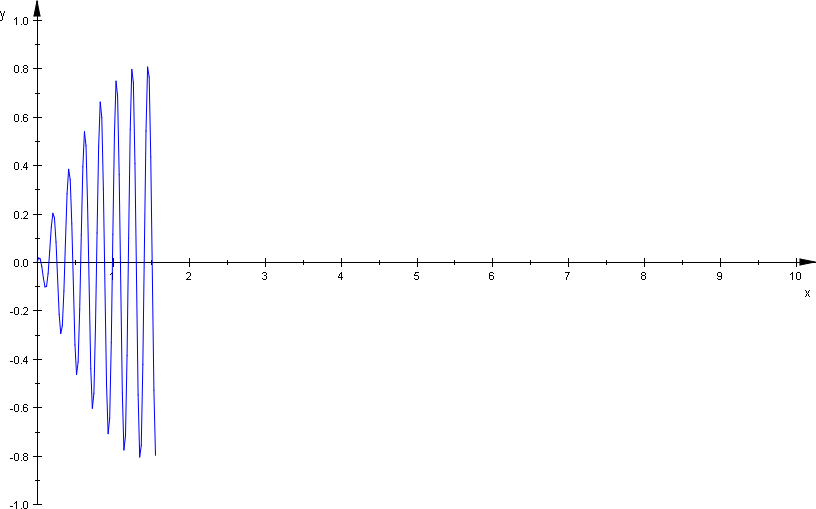Figure 2.12 — Left: Oscillations of diminishing amplitude in the primary (ring-down). Right: and oscillations of rising amplitude in the primary (ring-up).

#### Bounces

All the energy is now in the secondary circuit. With an ideal spark gap, things would stop here and a new cycle could begin. But this is of course not the case : the ring-up is very fast and the path of ionised air in the spark gap subsists a few moments even when the intensity of the field has fallen below the critical value. The energy of the secondary can therefore be retransmitted to the primary in a similar fashion. Current and voltage in the secondary will then diminish while those in the primary will increase.

Such bounces can occur 3, 4, 5 times or even more. At each rebound, a fraction of the energy is definitively lost, mainly in the sparks produced and in the internal resistances of the components. This is the reason why the envelop of the waveform falls exponentially. After a certain number of bounces, voltage will have decreased significantly and the spark gap finally opens at the next primary notch.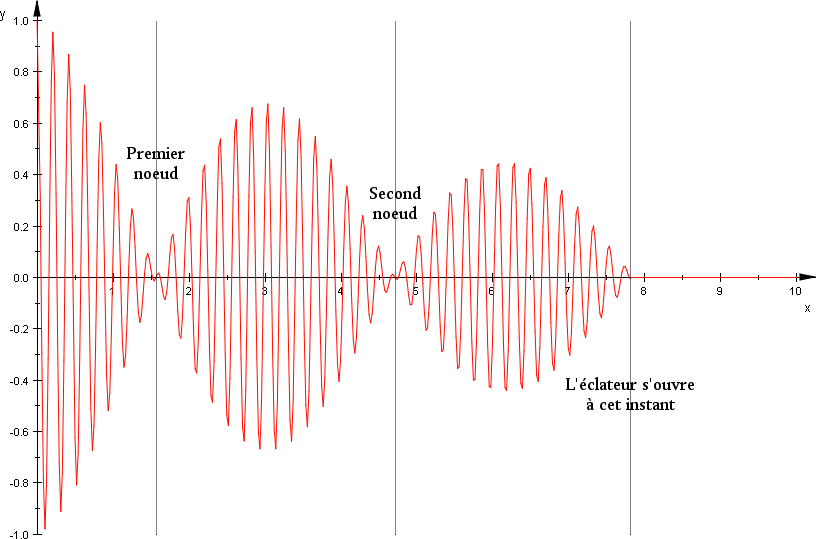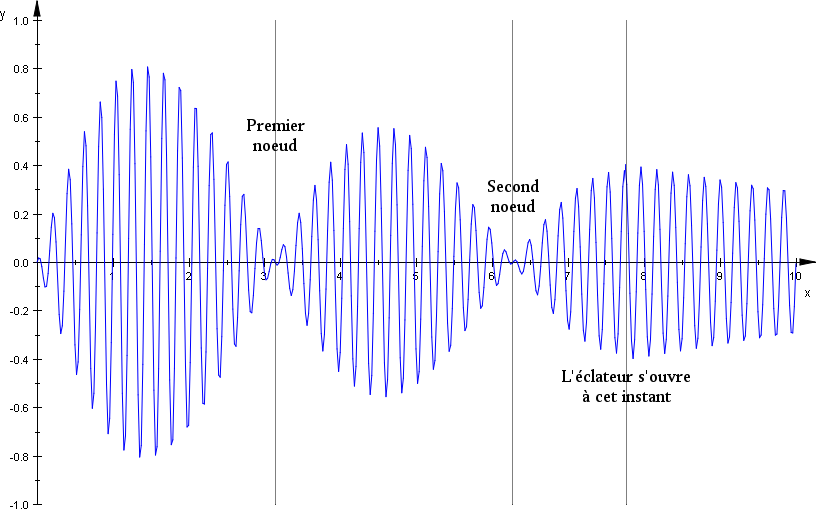Figure 2.13 — General waveform of the oscillation in the primary and secondary circuits. Here a cycle with 3 rebounds has been shown.

These rebounds are actually important for the creation of long arcs, because arcs grow on the ionised air path created during the previous rebounds. At each bounce, the spark gets longer. The complete process happens several hundred times per second.

#### Decay

Once the main spark gap has stopped firing, the primary circuit is open and all the remaining energy is trapped in the secondary. This situation is thus the same as in a free RLC circuit. Oscillations will decay exponentially as the charge dissipates through the sparks.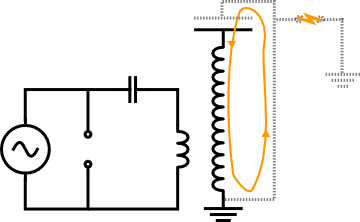Figure 2.14 — When the spark gap has opened, secondary oscillation will decay exponentially. Finally, a new cycle can begin.

### 2.4.2   Voltage gain

We will now derive a rough formula giving the secondary voltage Vout. We call Vin the rms voltage supplied by the transformer. The following derivation is based on conservation of energy (originally found here and here), we'll suppose there's no ohmic losses (R = 0) and that the primary and secondary circuits are perfectly at resonance. Let's recall the formula giving the energy stored in a capacitor as a function of the voltage E = CV2/2. The energy of the primary Ep circuit is thus given by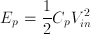where Cp is the capacity of the primary circuit. The energy Es stored in the secondary circuit (capacity is Cs) is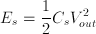Using the hypothesis that all the energy stored in the primary goes into the secondary, we equate Es and Ep :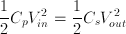The voltage gain is then:It is more convenient to express this gain in terms of the inductance of the circuits. Given the fact these are at resonance, we have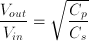The gain can therefore be rewritten this way: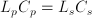Now we can understand how the Tesla coil can reach such tremendous voltages : the secondary coil, which has around 1000 turns, has an inductance considerably higher than the primary coil, which generally has about 10 turns. Using typical values, this formula will yield a Vout of the order of 105 V or even 106 V for the largest coils.

#### Energy losses

We supposed in the above derivation that energy losses are nonexistent. This is of course an approximation and the formula we found is then a upper bound. We'll describe here the major causes of these losses (source). These will be calculated/examined deeper in next page.

• The wiring and the inductances have an internal resistance that dissipates energy as heat following P = R I2. There are several hundred meters of wire in a Tesla coil. The skin effect, which accounts for the fact that at higher frequencies, the current flows mainly near the surface of the conductors, further increases the effective resistance.
• The sparks that occur in the spark gap act like a resistor, dissipating energy in the form of light, heat and sound.
• The dielectric inside the primary capacitor dissipates a fraction of the energy when an alternating current is applied. The loss tangent depends on the dielectric used and the frequency.
• The Tesla coil operates at radio frequencies (typically between 50 and 400 kHz). A fraction of the energy is thus radiated as electromagnetic waves.
• The Corona effect is a continuous discharge from the conductors in the ambient medium, producing a violet halo around conductors kept at high voltages. The energy used for ionising the air is taken away form the coil.

Let's finally note that primary and secondary circuits are rarely at perfect resonance, which contributes to a further reduction of the secondary voltage.

### 2.4.3   Distribution of capacitance within the secondary circuit

We will not examine the question of the secondary capacitance which we called Cs in previous discussions. This capacitance consists of many contributions and is difficult to compute, but we'll look at its major components (see figure below).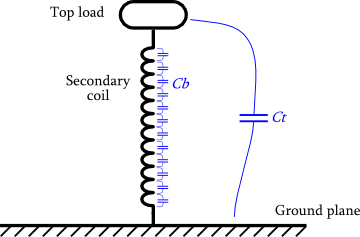Figure 2.15 — The major contributions to the secondary capacitance Cs.

Top load - Ground.   The highest fraction of the secondary capacitance comes from the top load. Indeed we have a capacitor whose "plates" are the top load and the ground. The dielectric is simply air. It might be surprising that this is indeed a capacitor as these plates are connected though the secondary coil. However, its impedance is quite high so there's actually quite a potential difference between them.

We shall call Ct this contribution.

Turns of the secondary coil.   The other big contribution comes from the secondary coil. It is made of many adjacent turns of enamelled copper wire and its inductance/resistance is therefore distributed along its length. This implies there's a slight potential difference between two adjacent turns. We then have two conductors at different potential, separated by a dielectric : that is a capacitor. Actually, there is a capacitor with every pair of wires, but its capacity decreases with distance, therefore one can consider the capacity only between two adjacent turns a good approximation.

Let's call Cb the total capacity of the secondary coil.

Actually, it's not mandatory to have a top load on a Tesla coil, as every secondary coil will possess its own capacity. We'll see however that a top load is crucial for having beautiful sparks.

Other contributions.   When a spark occurs at the top load, it will add a little extra capacity to the secondary circuit. This is most noticeable only on large coils however, whose spark are longer than a meter. This contribution will be negligible for our coil Zeus. Moreover, if the coil does not run on a verdant hill (which is often the case), there will be extra capacity form the surrounding objects. This capacitor is formed by the top load on one side and conducting objects (walls, plumbing pipes, furniture, etc.) on the other side. Actually there's even more sources of capacity (for example these of the "top load - primary coil" capacitor), but these really become negligible.

We'll name the capacitor of these external factors Ce.

The whole thing...   As all these "capacitors" are in parallel, so the total capacity of the secondary circuit will be given by :

Cs = Ct + Cb + Ce

Let's note that the reason why this capacity is so hard to define is because it is truly small (a few dozens of picoFarads). That's why all the factors must be taken in account. Indeed, they would also apply for the capacity of the primary circuit, but this one has a much greater capacity, which is totally concentrated in the primary capacitor, so the aforementioned factors are truly negligible.

### 2.4.4   Influence of the coupling

The constant of magnetic coupling k accounts for the fraction of the total field that the two coils are sharing. This constant is mainly determined by the relative position of the coil as well as their shape. We'll now see that this constant must be well-chosen.

Figure 2.16 — Waveforms of the primary and secondary voltage for increasing values of k. [Image credit : R. Burnett]

If the coupling is weak (k ≈ 0.05), a significant number of oscillations will be required for all the energy to be transferred to the secondary. The primary notches are attained in a smoother way, which allows the spark gap to open more easily at the first notch, or at least at early ones. This is a good thing as the amplitude of the envelop is decreasing and so does the energy of the system. However, if the first notch is too far, a non-negligible fraction of energy will be lost.

If the coupling is stronger (k ≈ 0.2), the number of oscillations required to transfer all the energy into the secondary will be lower. The rise of the voltage in the secondary coil is thus more brutal, which can cause arcs between the turns, a very bad thing. It will also be more difficult for the spark gap to open at the earliest notches (a powerful quenching system will be required). The advantage of strong coupling is that, if the aforementioned problems can be prevented, energy losses will be lower.

The ideal coupling is unique to each coil. Considering the above discussion, it is clear that high coupling constant will be preferable on coil of lower power. It's indeed unlikely that turn-shorting problems will occur as the overall voltages are lower. For the same reasons, a low coupling is recommended for high-power coils. It has also been suggested to avoid coupling between 0.43 and 0.53 (source).

### 2.4.5   A few words on the three-coil transmitter

At the beginning of this document, we mentioned an advanced version of the Tesla coil, which included three coils instead of two. The primary circuit will remain roughly the same, the main differences appear in the secondary inductor. The latter is actually divided into two parts : the "proper" secondary coil, which has an unusually high coupling with the primary (k ≈ 0.6) ; and an extra coil, the "magnifier", placed away from the magnetic field generated at the primary.Figure 2.17 — Configuration of the inductors on a magnifying transmitter.

In this design, the voltage is first raised from the primary to the secondary coil by magnetic induction, in a similar fashion as in a conventional transformer. The voltage is then further augmented by the magnifier, using resonant amplification (the top load is at the end of this coil), just like an LC circuit amplifies the signal it receives when at resonance. The idea here is to intensify the rise of the voltage by conceiving a more efficient way to use the emf generated in the secondary by the primary's magnetic field. This two-stage system can be seen as an hybrid between a classical Tesla coil and an induction transformer. This configuration is however more difficult to realise as a strong coupling between the first stage of the secondary and the primary must be realised by ensuring no arcs will occur between the coils. The apparatus' behaviour also seems to be more difficult to predict (more info on this website by Jamie Oliver).

## 2.5   Comparison with other devices

### 2.5.1   The induction transformer

The Tesla coil operates in a significantly different manner than does the induction transformer. Nevertheless the two apparatuses share interesting similarities, that is why we'll proceed though a quick comparison.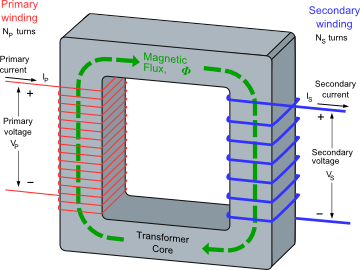Figure 2.18 — Left: Schematic of a basic induction transformer (here a step-down). Right: A real-life transformer. The wire of the primary is black, those of the secondary are green (the middle wire is the ground wire). [Image credit : Wikipedia]

In an induction transformer, the voltage and current are transformed by a pair of coils, also called primary and secondary, that are tightly wound around a core made of a highly permeable material. When alternating current arrives in the primary coil, an induced magnetic field appears, also alternating. This field will be considerably amplified by the core. As the secondary is also wound around this core, the aforementioned alternating magnetic field will induce an emf in the secondary. The latter provokes the apparition of a current, whose frequency is the same as the input current. For this kind of transformer, one can show that: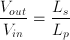The major difference with the Tesla coil is the absence of a capacitor in a induction transformer. (Well actually, capacitors can be found, in such transformers, for power factor correction for example, but these are not necessary for the transformation itself.) While the Tesla coils uses resonant amplification for increasing the voltage, the conventional transformer only uses magnetic induction. On the latter, primary and secondary coils have a very high coupling constant (as close to 1 as possible), while those of the Tesla coil share a coupling constant of much lower value, which can be directly seen in the disposition and shapes of the inductors and in the fact that they are air-cored. This explains why the gain in a conventional transformer is higher for given Lp and Ls (compare the Vout/Vin formulas). This is however not by chance that both the devices rely on coils of different inductance to transform a current. The point in a Tesla coil is that LpCp = LsCs must be achieved for efficient transformation.

Finally, let's note the absence of cycles in the operation of a induction transformer : the current is transformed directly. Moreover, a conventional transformer works at the frequency of the input current (within a certain "reasonable" range of frequencies), and the output current has the same frequency. But on a Tesla coil, the output current frequency is fixed.

### 2.5.2   The quarter-wave antenna

When an alternating current flows in a conductor, it generates an oscillating electromagnetic field which propagates like a wave. These radio waves are the basis of all telecommunications. It has been suggested that, by some aspects, a Tesla coil resembles a quarter-wave antenna. Actually, this model fails to describe the behaviour of a the Tesla coil in a satisfying way. It is moreover not necessary to tune the impedance of the coil like a quarter-wave antenna. Nevertheless, the common traits of these two devices are interesting enough to justify this short exposition.

Let's begin with this quick definition : a quarter-wave antenna is an antenna whose length L is equal to λ/4, where λ is the wavelength of the input signal. (Note that voltage as well as current are alternating signals, defining a wavelength thus makes sense.)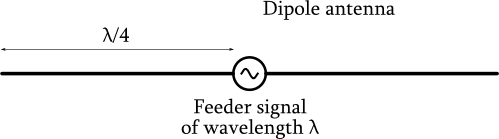Figure 2.19 — Basic schematic of a centre-fed, dipole antenna, its length is λ/2. Each branch can be seen as a quarter-wave antenna.

#### Comparison with the Tesla coil

One can compare the secondary circuit to one of the branches of a dipole antenna, the other branch being the ground.Figure 2.20 — Analogy between a quarter-wave antenna and the secondary circuit of a Tesla coil.

When a wave of wavelength λ reaches the extremity of the antenna, it will be totally reflected. One can show that if the length of the antenna (which would correspond to the length of the wire used in the secondary winding of the Tesla coil) is an integer multiple of λ/4, this reflexion will cause stationary waves to appear. In other words, its length L must be

L = nλ/4,      with n = 1, 2, 3, ...

On a quarter-wave, n is equal to 1. Let's examine the nature of the stationary wave for the current I(x,t) and voltage E(x,t). One can show that if n is odd, then there will be a voltage node at the beginning of the antenna and a voltage antinode at its extremity. For the current, there will be an antinode at the base of the antenna and a node at its extremity. In other words,

E(0,t) = 0

E(L,t) = E0cos(ωt)

and

I(0,t) = I0cos(ωt)

I(L,t) = 0

where E0 and I0 denote the maximal amplitude of the wave, and ω = λ/c, where c is the speed of propagation of electromagnetic waves (about 3·108 m/s). We've chosen the origin of times so there is no phase.

Figure 2.21 — Behaviour of the voltage E(x,t) and current I(x,t) on an antenna of length λ/4 (left) and 3λ/4 (right). [Image credits: Adapted from All About Circuits]

We can thus easily understand the benefits that we could, theoretically, have by tuning a Tesla coil following the quarter-wave antenna model, i.e. choosing the length of the secondary wire such as it is one quarter of the wavelength at resonant frequency. This would indeed ensure there is an antinode of voltage at the top of the coil, which is what we want. However, it has been experimentally demonstrated that the currents at the base and at the top of the coil are actually almost in phase (source). This shows the quarter-wave antenna model doesn't apply for the Tesla coil and that one can consider its capacitance and inductance to be lumped.

## 2.6   Analysis of two coupled circuits

We're about to analyse the behaviour of a pair of inductively coupled LC and RLC circuit, the former being identical to an ideal Tesla coil with spark gap closed. The self-inductance and capacitance of the primary circuit are respectively L1 and C1 while those of the secondary circuit are L2 and C2. The mutual inductance between the circuits is denoted M.

For both cases I've used Mathematica to solve the systems of equations. If you feel like playing with the programmes to see how solutions behave, feel free to download them using the links on the right.

### 2.6.1   Two inductively coupled LC circuitsTwo inductively coupled LC circuits describes an ideal, lossless Tesla coil. The circuit we'll deal with are shown on the right. Kirchoff's law for current tells that the current is identical in the primary loop, we'll note it I1. Same thing with the current flowing within the secondary loop, I2. Note that, rigorously speaking, we know that the conventional Kirchoff's laws won't apply here, as the dimensions of the secondary circuit is not negligible comparing to the signal wavelength, but the results we'll derive, assuming they hold, will nevertheless give a good idea of what's happening in a Tesla coil.

Using the differential expressions of the components, the equation system describing the present circuit is the following :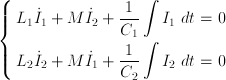which we rewrite in a nicer form: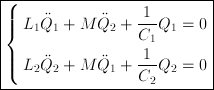We'll resolve this system with the following initial conditions :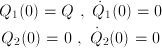which correspond to the situation where only the primary capacitor is charged (Q = C1V, without any other charge or current elsewhere in the circuits, just like in a Tesla coil). Solving the system, we get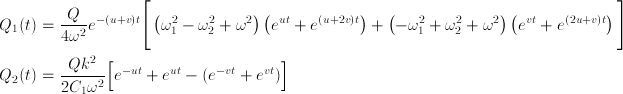where we've replaced the following quantities :k is the coupling constant binding the primary and secondary circuits (we've talked about it earlier) ; we immediately check it is a dimensionless quantity. It can be shown that :

0 < k < 1

The case k = 0 is meaningless/irrelevant here as it implies the two circuits aren't interacting and the case k = 1 is never physically attained.

ω1 and ω2 respectively represent the resonant pulsation of the primary and secondary circuit. ω has thus also the dimensions of a pulsation. Moreover, because of the above condition,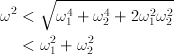u et v also have the dimensions of a pulsation. We can also see they are actually purely imaginary quantities. Indeed, the numerator is positive and both constant while the denominator is always negative (because of the conditions on k again). We can thus rewrite as follows :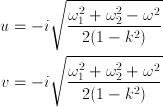We therefore deduce the expression for Q1 and Q2 to be oscillating solutions, as sums of imaginary exponentials.

#### Solution for ω1 = ω2

We've made no hypothesis on the circuits so far. We'll now look at the case when both circuits are at resonance, just as in a Tesla coil. When ω1 = ω2, the functions obtained become less gory and easier to physically interpret. We thus get :The solutions previously obtained for Q1 and Q2 then become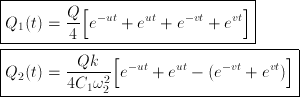If coupling is weak, i.e. k ≪ 1,is close to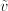and we have (-) ≪ (+). We can easily see that solutions obtained both represents beats. Indeed, applying Euler's formula, we get :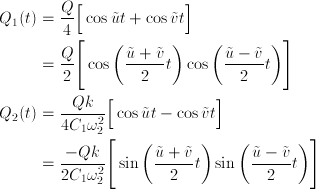The following plots of Q1 and Q2 indeed correspond to what we expected. The only difference with the real case is the absence of exponential decrease of the envelop. This is because we considered LC circuits, where resistance is zero.Figure 2.22 — Plots of Q1(t) and Q2(t) for two inductively coupled LC circuits
with Q = 1, ω1 = ω2 = 0.5, M/L1 = 0.05, and M/L2 = 0.025.

### 2.6.2   Two inductively coupled RLC circuitsNow we consider the resistance of both circuits to be non-zero and represent it by a lumped resistor in series. Two inductively coupled RLC circuits is a much more accurate description of a Tesla coil. The circuit we'll deal with are shown on the right. Like previously, we call I1 the current flowing in the primary circuit and I2 the one flowing in the secondary.

Using the differential expression of the component, the equation system describing this circuit is the following :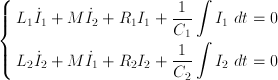or, in term of the charge in the capacitors :Actually, this system has no analytic solution in the general case (see Marco Denicolai, Optimal performances for tesla transformers for more details), even when we consider the circuits to be at resonance. The system thus has to be resolved numerically. After implementing the aforementioned system in a computing software with the same initial conditions as before, we get the functions that have been plotted in the next figure.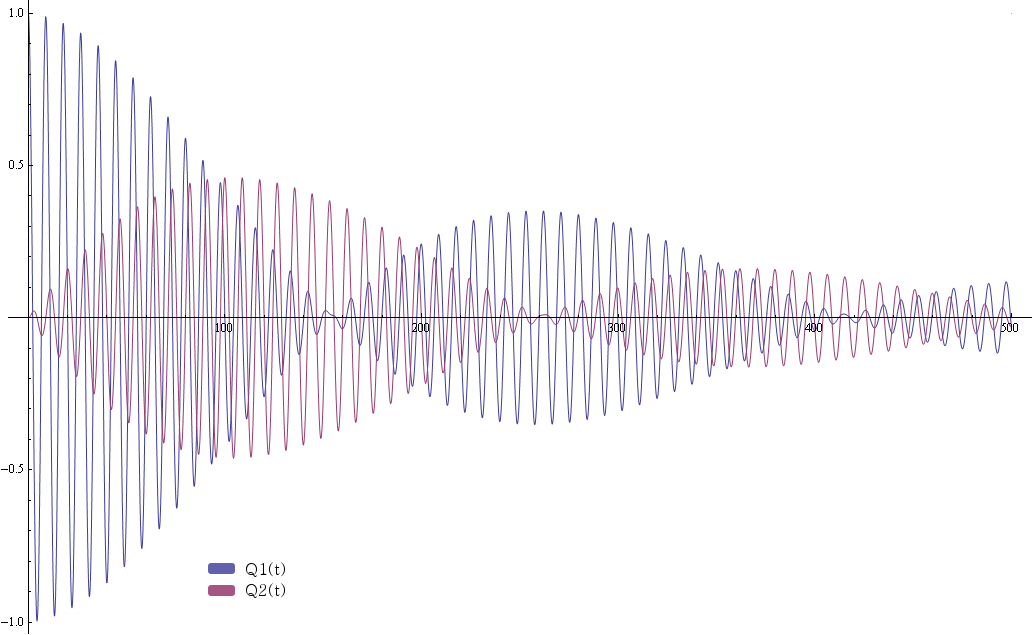Figure 2.23 — Plots of Q1(t) and Q2(t) for two inductively coupled RLC circuits
with Q = 1, ω1 = ω2 = 0.5, M/L1 = 0.05, M/L2 = 0.025, R1/L1 = 0.001, R2/L2 = 0.015.

This kind of graph was exactly what we expected. The influence of the resistors has been exaggerated in order to emphasise their effects. We thus see that, beside the exponential decrease of the envelops, the new terms in the first time-derivative of the charge deform the envelops shape and also shift them with respect to each other : the primary nodes no longer exactly coincide with the secondary antinodes and vice-versa. The shift between the secondary node and the primary antinode is however less obvious because we considered the primary resistance to be much lower than the secondary. In a real Tesla coil, much of the energy dissipation indeed occurs within the secondary.

That's it for the theory of operation ! We now know how and why this machine is able generate such great sparks. Time to move on to the actual building of a Tesla coil.